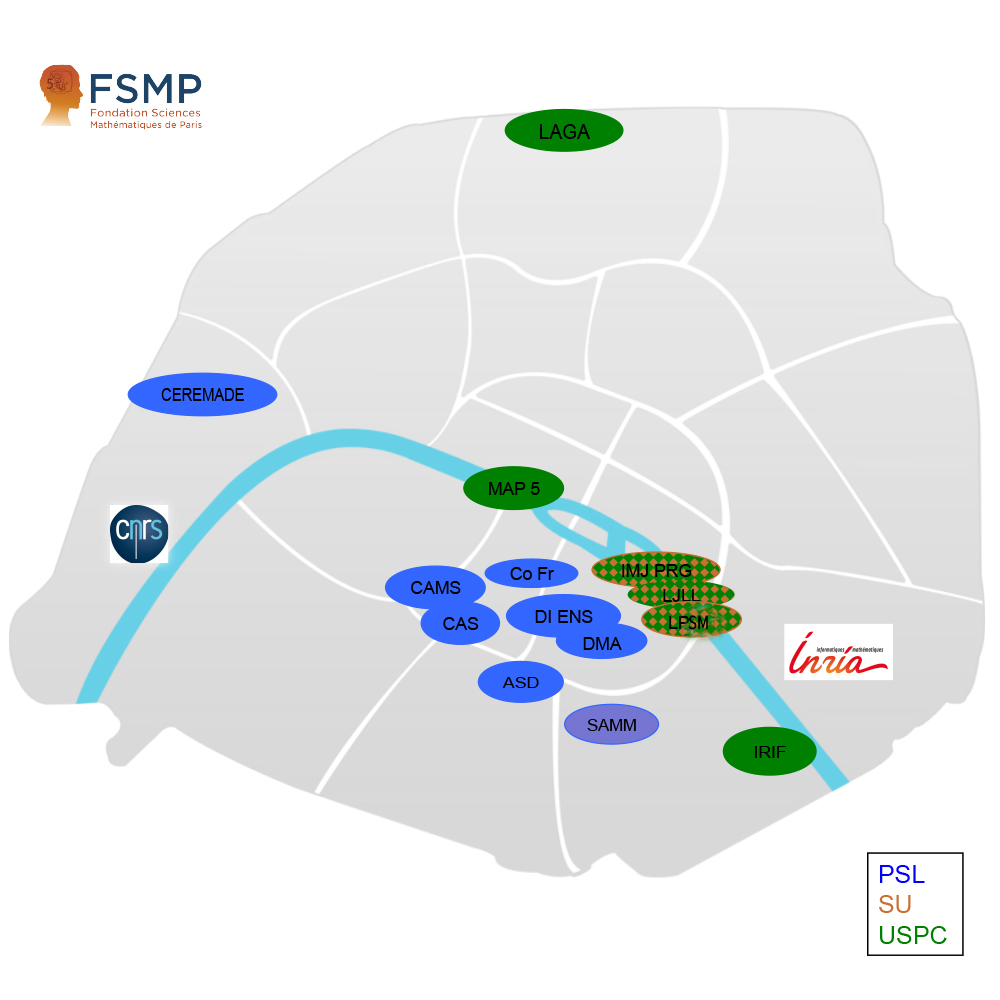# How to find an PhD advisor or a Post-doc supervisor ?

### Recevez la lettre d'information

Télécharger la lettre d'information

### Our laboratoriesWe have scientific contacts in many countries where maths studies are developed.

If you need any dedicated advice with one of our ambassadors in your country, you can contact us at mathinparis - at - fsmp - dot - fr

Fondation Sciences Mathématiques de Paris (FSMP) is an excellence network in mathematics and fundamental computer science in Paris, founded by CNRS, Sorbonne University (SU), Université de Paris and the 4 Mathematics Chairs of Collège de France. Partners are Inria, Université Paris-Dauphine, Paris 1 Panthéon-Sorbonne, Université Sorbonne Paris-Nord, and the IdEx PSL (teams of Ecole des Mines, Observatoire de Paris and EHESS.)

The FSMP is part of a research mathematical and computer science network bringing together 14 laboratories and 23 Inria teams in Paris belonging to three collegiate university :

Your research can be hosted in one of these laboratories or in one of the Inria teams. (The complete list is available here)

### Researchers in our laboratories mainly work on :

General Pure Mathematics

•    Algebra : DMA, IMJ-PRG
•    Algebraic Topology and Geometry : DMA, IMJ-PRG, INRIA, LAGA
•    Analysis and Operators Algebras: DMA, CEREMADE, IMJ-PRG, LJLL
•    Arithmetic-Number Theory : DMA, IMJ-PRG, LAGA
•    Combinatorics and Optimization : DI ENS, IMJ-PRG,  IRIF
•    Differential geometry : DMA, CEREMADE,  IMJ-PRG
•    Logic and Foundations : DMA, IMJ-PRG
•    Dynamical systems, Ordinary Differential Equation and applications : ASD, CAMS, CEREMADE, DMA, IMJ-PRG, LAGA, LPSM

History of Mathematics :  CAMS, IMJ-PRG

General Applied Mathematics•

• Applied Functional Analysis : CEREMADE, LJLL, SAMM
• Non linear analysis and Partial Differential Equation :  CAMS, CEREMADE, DMA, IMJ-PRG, INRIA, LAGA, LJLL, SAMM
• Mathematical optimization, optimal control theory and applications : CEREMADE, DMA, IMJ-PRG, INRIA, LJLL, SAMM
• Numerical analysis : CEREMADE, INRIA, LJLL

Probability, statistics and applications

•  Probability : CEREMADE, DMA, LAGA, LPSM, MAP5, SAMM
•  Statistics : CEREMADE, DMA, INRIA, LAGA, LPSM, MAP5, SAMM
•  Biostatistics :  DMA, CEREMADE, INRIA, LPSM
• Actuarial science : CEREMADE, LPSM
• Mathematical Finance : CEREMADE, INRIA, LAGA,  LPSM

Mathematical/Computational modeling

• Mathematical image processing and data analysis: CEREMADE, CAMS, DMA, INRIA, LAGA, LJLL, MAP5
• Mathematical modeling applied to medicine and life science : CEREMADE, DMA, INRIA, IRIF, LJLL, LAGA, LPSM, MAP5
• Mathematical modeling for physics, chemistry and mechanics : CEREMADE,  INRIA, IMJ-PRG, LJLL, LAGA, LPSM, MAP5
• Mathematical modeling for complex systems in social sciences : CAMS, CEREMADE, INRIA, LJLL, IRIF
• Mathematical modeling for optimization, control theory and Mean Field Games: DMA, CEREMADE, INRIA, LJLL

Computer Science

• Discrete structures, graph theory, randomness and geometry : DI-ENS, INRIA, IMJ-PRG, IRIF, LPSM
• Logic and verification, Proof theory, Verification by model checking, Database theory, design and models : DI-ENS, INRIA, IRIF
• Programming language theory, Software and its engineering : INRIA, IRIF
• Design and analysis of algorithms, Computer graphics : DI-ENS, INRIA
• Security and privacy, Cryptography : DI-ENS, INRIA,
• Quantum computing : INRIA, IRIF
• Computational biology and Bioinformatics : INRIA, IRIF

For more information you can write to : mathinparis - at - fsmp - dot - fr